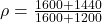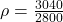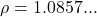## 1600cm of fresh water of density 1g/cm are mixed with 1200 cm of sea water of density 1.2g/cm’. Determine the density of the mi

Question

1600cm of fresh water of density 1g/cm are mixed with 1200 cm of sea water of
density 1.2g/cm’. Determine the density of the mixture..

in progress 0
3 months 2021-08-12T23:56:34+00:00 2 Answers 8 views 0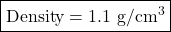Explanation:

Volume of fresh water:

Volume = Mass / Density

Volume = 1600/1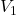= 1600 cm³

Volume of sea water:

Volume = Mass / Density

Volume = 1200/1.2

Volume = 1000 cm³

So,

Total Volume when they are mixed = 1600+1000

Total Volume when they are mixed = 2600 cm³

And,

Total Mass when they are mixed = 1600+1200

Total Mass when they are mixed = 2800 g

Now, Measuring the density of the mixture:

Density = Mass / Volume

Density = 2800/2600

Density = 1.1 g/cm³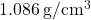Explanation:

Volume of fresh water ⇒ 1600 cm³

Density of fresh water ⇒ 1 g/cm³

Volume of sea water ⇒ 1200 cm³

Density of sea water ⇒ 1.2 g/cm³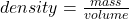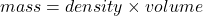Calculate mass of fresh water.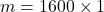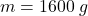Calculate the mass of sea water.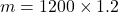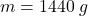Find the density of the mixture.